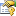﻿ ProbabilisticCoordinateDescent(TKernel, TInput) ClassL1-regularized logistic regression (probabilistic SVM) learning algorithm (-s 6).Inheritance Hierarchy
SystemObject
Accord.MachineLearningBinaryLearningBaseSupportVectorMachineTKernel, TInput, TInput
Accord.MachineLearning.VectorMachines.LearningBaseSupportVectorClassificationSupportVectorMachineTKernel, TInput, TKernel, TInput
Accord.MachineLearning.VectorMachines.LearningBaseProbabilisticCoordinateDescentSupportVectorMachineTKernel, TInput, TKernel, TInput
Accord.MachineLearning.VectorMachines.LearningProbabilisticCoordinateDescentTKernel, TInput

Namespace:  Accord.MachineLearning.VectorMachines.Learning
Assembly:  Accord.MachineLearning (in Accord.MachineLearning.dll) Version: 3.8.0Syntax
public class ProbabilisticCoordinateDescent<TKernel, TInput> : BaseProbabilisticCoordinateDescent<SupportVectorMachine<TKernel, TInput>, TKernel, TInput>
where TKernel : struct, new(), ILinear<TInput>


#### Type Parameters

TKernel
TInput

The ProbabilisticCoordinateDescentTKernel, TInput type exposes the following members.Constructors
NameDescriptionProbabilisticCoordinateDescentTKernel, TInput
Constructs a new Newton method algorithm for L1-regularized logistic regression (probabilistic linear vector machine).
TopProperties
NameDescriptionC
Gets or sets the cost values associated with each input vector.
(Inherited from BaseSupportVectorClassificationTModel, TKernel, TInput.)Complexity
Complexity (cost) parameter C. Increasing the value of C forces the creation of a more accurate model that may not generalize well. If this value is not set and UseComplexityHeuristic is set to true, the framework will automatically guess a value for C. If this value is manually set to something else, then UseComplexityHeuristic will be automatically disabled and the given value will be used instead.
(Inherited from BaseSupportVectorClassificationTModel, TKernel, TInput.)Inputs
Gets or sets the input vectors for training.
(Inherited from BaseSupportVectorClassificationTModel, TKernel, TInput.)Kernel
Gets or sets the kernel function use to create a kernel Support Vector Machine. If this property is set, UseKernelEstimation will be set to false.
(Inherited from BaseSupportVectorClassificationTModel, TKernel, TInput.)MaximumIterations
Gets or sets the maximum number of iterations that should be performed until the algorithm stops. Default is 1000.
(Inherited from BaseProbabilisticCoordinateDescentTModel, TKernel, TInput.)MaximumLineSearches
Gets or sets the maximum number of line searches that can be performed per iteration. Default is 20.
(Inherited from BaseProbabilisticCoordinateDescentTModel, TKernel, TInput.)MaximumNewtonIterations
Gets or sets the maximum number of inner iterations that can be performed by the inner solver algorithm. Default is 100.
(Inherited from BaseProbabilisticCoordinateDescentTModel, TKernel, TInput.)Model
Gets or sets the classifier being learned.
(Inherited from BinaryLearningBaseTModel, TInput.)NegativeWeight
Gets or sets the negative class weight. This should be a value higher than 0 indicating how much of the Complexity parameter C should be applied to instances carrying the negative label.
(Inherited from BaseSupportVectorClassificationTModel, TKernel, TInput.)Outputs
Gets or sets the output labels for each training vector.
(Inherited from BaseSupportVectorClassificationTModel, TKernel, TInput.)PositiveWeight
Gets or sets the positive class weight. This should be a value higher than 0 indicating how much of the Complexity parameter C should be applied to instances carrying the positive label.
(Inherited from BaseSupportVectorClassificationTModel, TKernel, TInput.)Token
Gets or sets a cancellation token that can be used to stop the learning algorithm while it is running.
(Inherited from BinaryLearningBaseTModel, TInput.)Tolerance
Convergence tolerance. Default value is 0.01.
(Inherited from BaseProbabilisticCoordinateDescentTModel, TKernel, TInput.)UseClassProportions
Gets or sets a value indicating whether the weight ratio to be used between Complexity values for negative and positive instances should be computed automatically from the data proportions. Default is false.
(Inherited from BaseSupportVectorClassificationTModel, TKernel, TInput.)UseComplexityHeuristic
Gets or sets a value indicating whether the Complexity parameter C should be computed automatically by employing an heuristic rule. Default is true.
(Inherited from BaseSupportVectorClassificationTModel, TKernel, TInput.)UseKernelEstimation
Gets or sets whether initial values for some kernel parameters should be estimated from the data, if possible. Default is true.
(Inherited from BaseSupportVectorClassificationTModel, TKernel, TInput.)WeightRatio
Gets or sets the weight ratio between positive and negative class weights. This ratio controls how much of the Complexity parameter C should be applied to the positive class.
(Inherited from BaseSupportVectorClassificationTModel, TKernel, TInput.)
TopMethods
NameDescriptionComputeError Obsolete.
Computes the error rate for a given set of input and outputs.
(Inherited from BaseSupportVectorClassificationTModel, TKernel, TInput.)Create
Creates an instance of the model to be learned. Inheritors of this abstract class must define this method so new models can be created from the training data.
(Overrides BaseSupportVectorClassificationTModel, TKernel, TInputCreate(Int32, TKernel).)Equals
Determines whether the specified object is equal to the current object.
(Inherited from Object.)Finalize
Allows an object to try to free resources and perform other cleanup operations before it is reclaimed by garbage collection.
(Inherited from Object.)GetHashCode
Serves as the default hash function.
(Inherited from Object.)GetType
Gets the Type of the current instance.
(Inherited from Object.)InnerRun
Runs the learning algorithm.
(Inherited from BaseProbabilisticCoordinateDescentTModel, TKernel, TInput.)Learn(TInput, Boolean, Double)
Learns a model that can map the given inputs to the given outputs.
(Inherited from BinaryLearningBaseTModel, TInput.)Learn(TInput, Double, Double)
Learns a model that can map the given inputs to the given outputs.
(Inherited from BinaryLearningBaseTModel, TInput.)Learn(TInput, Int32, Double)
Learns a model that can map the given inputs to the given outputs.
(Inherited from BinaryLearningBaseTModel, TInput.)Learn(TInput, Int32, Double)
Learns a model that can map the given inputs to the given outputs.
(Inherited from BinaryLearningBaseTModel, TInput.)Learn(TInput, Boolean, Double)
Learns a model that can map the given inputs to the given outputs.
(Inherited from BaseSupportVectorClassificationTModel, TKernel, TInput.)MemberwiseClone
Creates a shallow copy of the current Object.
(Inherited from Object.)Run Obsolete.
Obsolete.
(Inherited from BaseSupportVectorClassificationTModel, TKernel, TInput.)Run(Boolean) Obsolete.
Obsolete.
(Inherited from BaseSupportVectorClassificationTModel, TKernel, TInput.)ToString
Returns a string that represents the current object.
(Inherited from Object.)
TopExtension Methods
NameDescriptionHasMethod
Checks whether an object implements a method with the given name.
(Defined by ExtensionMethods.)IsEqual
Compares two objects for equality, performing an elementwise comparison if the elements are vectors or matrices.
(Defined by Matrix.)To(Type)Overloaded.
Converts an object into another type, irrespective of whether the conversion can be done at compile time or not. This can be used to convert generic types to numeric types during runtime.
(Defined by ExtensionMethods.)ToTOverloaded.
Converts an object into another type, irrespective of whether the conversion can be done at compile time or not. This can be used to convert generic types to numeric types during runtime.
(Defined by ExtensionMethods.)
TopRemarks

This class implements a SupportVectorMachine learning algorithm specifically crafted for probabilistic linear machines only. It provides a L1- regularized coordinate descent learning algorithm for optimizing the learning problem. The code has been based on liblinear's method solve_l1r_lr method, whose original description is provided below.

Liblinear's solver -s 6: L1R_LR. A coordinate descent algorithm for L1-regularized logistic regression (probabilistic svm) problems.

min_w \sum |wj| + C \sum log(1+exp(-yi w^T xi)),

Given: x, y, Cp, Cn, and eps as the stopping tolerance

See Yuan et al. (2011) and appendix of LIBLINEAR paper, Fan et al. (2008)See Also